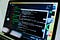Generic is a silent hero in C # .NET (Folk knew as hiding hero). Generic 1 in “5 superhero brothers” constitutes LINQ (the remaining 4 are: Extension method, Delegate, Lambda Expression and yield). He hides in 50% of the code we write, so much so that we unconsciously use it, not knowing his existence or his name.Photo by NESA by Makers on Unsplash

I wrote this article to honour the “silent hero” Generic, as well as guide you on how to create and use generic classes and generic methods.

# The birth of Generic

Many of us use generic without knowing that it is generic. For example, when we want to create a list of students, we often write:

`// A list of objects is StudentList <Student> students = new List <Student> ();students.Add (new Student ()); // The code is correctstudents.Add (new Car ()); // Compile error// Get the first student.// Compiler interprets itself as a StudentStudent first = students.First ();`

Yes, you will ask: Oh, this code is normal, do you see the hero hiding Generics? Yes, Generics hides inside those curly braces <>.

To make it easy to understand, let’s go back to .NET 1.0 when generics didn’t exist:

`// No generic// A list containing objectsList students = new List ();students.Add (new Student ()); // Normal compilestudents.Add (new Car ()); // Normal compile// Get the first object, must cast to StudentStudent first = (Student) students.First ();`

Without generics, the compiler cannot check errors at compiler time. Therefore, inline 2, we can add a Car object and a list of Student objects. When removing an element, we also have to cast it, because the compiler only understands it is an object. For these reasons, generic has been added in .NET 2.0. Effects of generic:

• Help reuse the code. For example, We just need to write class List <T>, There can be any class.
• Support compiler catch errors during compiler (Limiting the situation as line 2).
• No more casting from the object.

# Use Generic

Generic is applied in many classes List, Dictionary and LINQ, … When should we use LINQ? When we need to write a function that is reusable for many data types.

Sounds a bit confusing, isn’t it? Suppose I want you to write a swap function for 2 variables, can be used for types int, double, bool, including the class, how will you write?

Without generic

`public void swap (int ref a, int ref b);public void swap (double ref a, double ref b);public void swap (bool ref a, bool ref b);public void swap (object ref a, object ref b);......... // If you want to swap 2 students, you must write more functionspublic void swap (Student ref a, Student ref`

With the power of Generic, we only need to write 1 and only 1 method as follows:

`// Mark <T> to know the method is a generic methodpublic static void Swap <T> (ref T a, ref T b){   T temp = b;   b = a;   a = temp;}`

Using the generic is as follows:

`int firstInt = 1;int secondInt = 2;Swap <int> (ref firstInt, ref secondInt); double firstDouble = 1.5;double secondDouble = 2.5;// Can omit the <> because the compiler understands double// Based on the parameter we passedSwap (ref firstDouble, ref secondDouble);`

You can test the code here: https://dotnetfiddle.net/7zv1lM. Dotnet fiddle allows you to write and run C # code online, without any installation.

One more example, I just met in the process of code, it is used to get a random value in the list. We write a generic and use method (This method can be used with type int, double, … both Student or object, …).

`public static T getRandomElement <T> (List <T> list){    Random ran = new Random ();    int randomIndex = ran.Next (list.Count - 1);    return list [randomIndex];} //UseList <int> intList = new List <int> {1,2,3,4,5,6};List <double> doubleList = new List <double> {0.4, 0.6,0.8,4.5,0.2}; var randomInt = getRandomElement (intList);var randomDouble = getRandomElement (doubleList);`

Improved a bit by turning this method into an extension method for the List class:

`public static class ListExt{   public static T randomElement <T> (this List <T> list)   {     Random ran = new Random ();     int randomIndex = ran.Next (list.Count - 1);     return list [randomIndex];   }} //UseList <int> intList = new List <int> {1,2,3,4,5,6};List <double> doubleList = new List <double> {0.4, 0.6,0.8,4.5,0.2};// Extension methodConsole.WriteLine (intList.randomElement ());Console.WriteLine (doubleList.randomElement ());`

You can try this code here: https://dotnetfiddle.net/MZJ5HY

As introduced at the beginning of the article, Generic is a pretty powerful tool, can save code time, as well as minimize many bugs in your code. If you want to learn more, you can read more here: https://msdn.microsoft.com/en-us/library/512aeb7t.aspx. See you in the following article.

Always be nice to anybody who has access to my toothbrush.